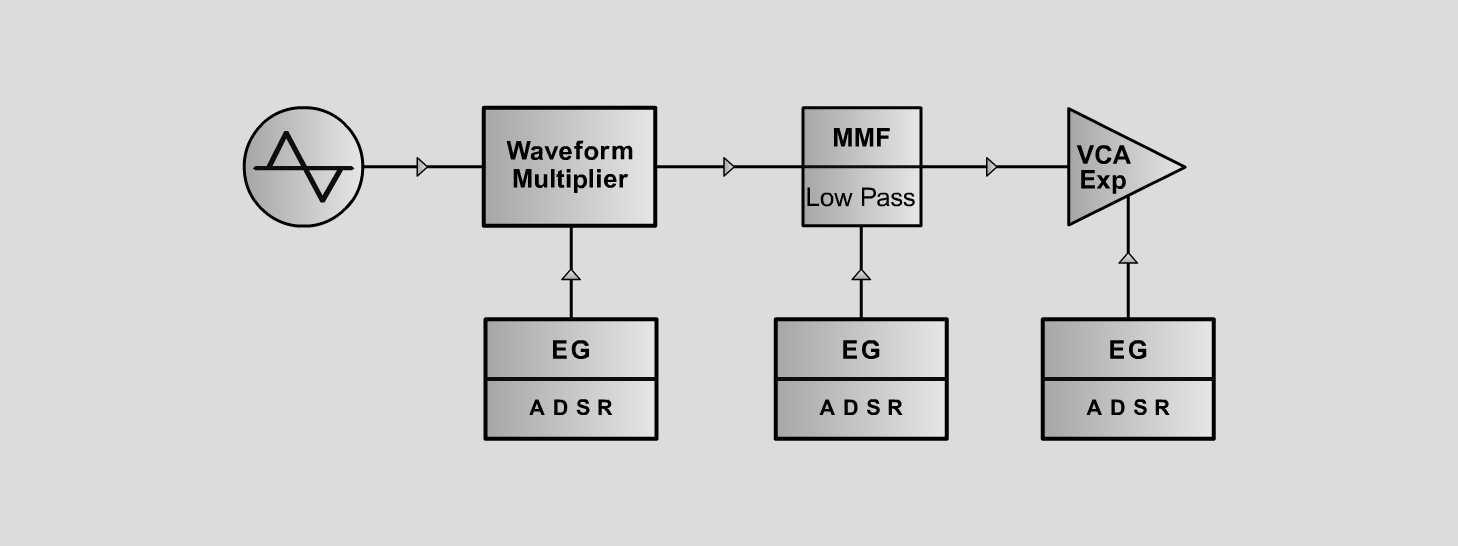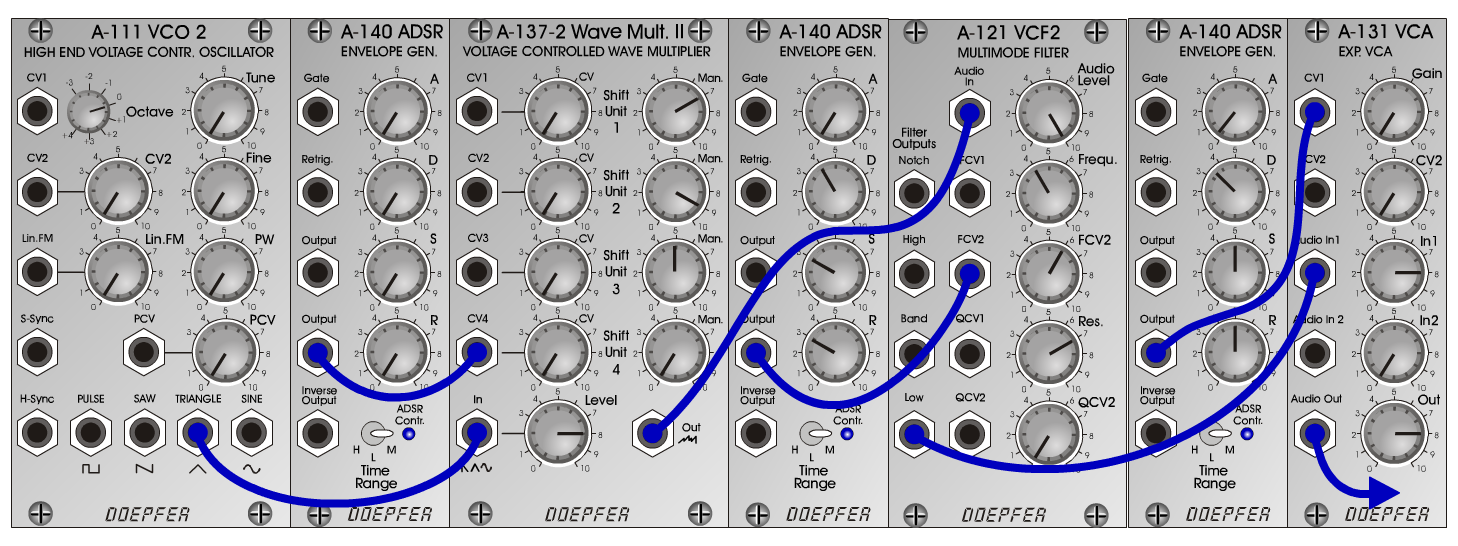TritonS y n t hD o e p f e r A - 100 Connections: Settings: Triton 0 A-111 (Tri) <=> A-137-2 (In)   A-137-2 (Out) <=> A-121 (Audio In)   A-121 (Low) <=> A-131 (Audio In)   A-140/1 (Output) <=> A-137-2 (CV4)   A-140/2 (Output) <=> A-121 (FCV2)   A-140/3 (Output) <=> A-131 (CV1) A-137-2 (Man 1 = 7, Man 2 = 9, Man 3 = 5, Man 4 = 0,   Level = 8)   A-121 (Audio Level = 10, Freq = 4, FCV 2 = 6, Res = 7)   A-131 (Gain = 0, Audio In 1 = 8, Audio Out = 8)   A-140/1 (A = 0, D = 0, S = 0, R = 0, Range = M)   A-140/2 (A = 0, D = 4, S = 3, R = 3, Range = M)   A-140/3 (A = 0.3, D = 3.5, S = 5, R = 5, Range = M) Triton 1 A-137-2 (Man 1 = 3, Man 2 = 6, Man 3 = 7, Man 4 = 5,   Level = 5)   A-121 (Audio Level = 8, Freq = 6, FCV 2 = 4, Res = 5)   A-131 (Gain = 0, Audio In 1 = 5, Audio Out = 5)   A-140/1 (A = 0, D = 0, S = 0, R = 0, Range = M)   A-140/2 (A = 0, D = 3, S = 2, R = 3, Range = M)   A-140/3 (A = 0, D = 4, S = 0, R = 2, Range = M) Triton 2 A-137-2 (Man1 = 4, Man 2 = 8, Man 3 = 3, Man 4 = 0, CV4 = 8,   Level = 5)   A-121 (Audio Level = 8, Freq = 6, FCV 2 = 2, Res = 4)   A-131 (Gain = 0, Audio In 1 = 5, Audio Out = 5)   A-140/1 (A = 0, D = 4, S = 0, R = 3, Range = M)   A-140/2 (A = 0, D = 2, S = 0, R = 2, Range = M)   A-140/3 (A = 0, D = 3, S = 0, R = 3, Range = M) Triton 3 A-137-2 (Man1 = 3, Man 2 = 7, Man 3 = 5, Man 4 = 5, CV4 = 1,   Level = 5)   A-121 (Audio Level = 8, Freq = 8, FCV 2 = 2, Res = 7)   A-131 (Gain = 0, Audio In 1 = 5, Audio Out = 5)   A-140/1 (A = 0, D = 4, S = 0, R = 3, Range = M)   A-140/2 (A = 0, D = 2, S = 0, R = 2, Range = M)   A-140/3 (A = 0, D = 3, S = 0, R = 3, Range = M) Josef MuellerSound samples Triton 0 (65 Herz) Triton 0 (131 Herz) Triton 0 (262 Herz) Triton 1 (65 Herz) Triton 1 (131 Herz) Triton 1 (262 Herz) Triton 2 (65 Herz) Triton 2 (131 Herz) Triton 2 (262 Herz) Triton 3 (65 Herz) Triton 3 (131 Herz) Triton 3 (262 Herz)